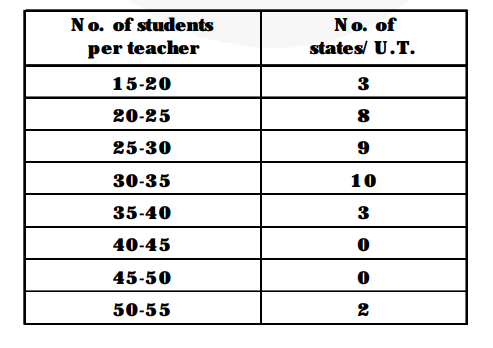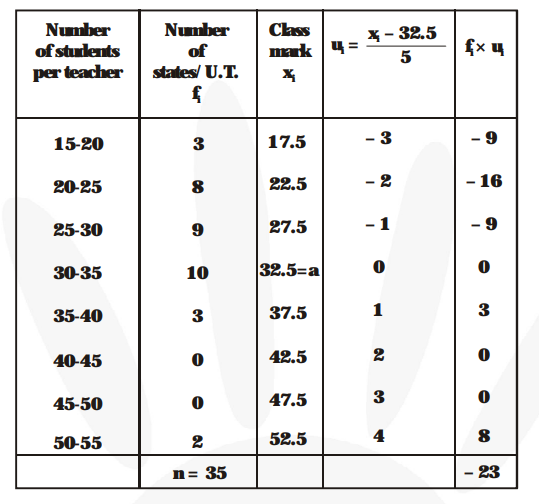# The following distribution gives the state-wise teacher-student ratio in higher secondary schools of India.

Question:

The following distribution gives the state-wise teacher-student ratio in higher secondary schools of India. Find the mode and mean of this data. Interpret, the two measures.Solution:

Modal class is (30-35) and its frequency is 10.

So, $\ell=30, \mathrm{f}_{\mathrm{m}}=10, \mathrm{f}_{1}=9, \mathrm{f}_{2}=3, \mathrm{~h}=5$.

Mode $=\ell+\left\{\frac{\mathbf{f}_{\mathbf{m}}-\mathbf{f}}{\mathbf{2 f}_{\mathbf{m}}-\mathbf{f}-\mathbf{f}_{2}}\right\} \times \mathbf{h}$

$=30+\left\{\frac{\mathbf{1 0}-\mathbf{9}}{\mathbf{2 0}-\mathbf{9}-\mathbf{3}}\right\} \times 5=30+\frac{\mathbf{5}}{\mathbf{8}}=30.6$$\mathrm{a}=32.5, \mathrm{~h}=5, \mathrm{n}=35$ and $\Sigma \mathrm{f}_{\mathrm{i}} \mathrm{u}_{\mathrm{i}}=-23$

By step-deviation method,

Mean $=\mathrm{a}+\mathrm{h} \times \frac{\mathbf{1}}{\mathbf{n}} \times \Sigma \mathrm{f}_{\mathrm{i}} \mathrm{u}_{\mathrm{i}}$

$=32.5+5 \times \frac{\mathbf{1}}{\mathbf{3 5}} \times(-23)$

$=32.5-\frac{\mathbf{2 3}}{\mathbf{7}}=32.5-3.3=29.2$

Hence, Mode = 30.6 and Mean = 29.2. We conclude that most states/U.T. have a student teacher ratio of 30.6 and on an average, the ratio is 29.2.